International
Tables for
Crystallography
Volume C
Mathematical, physical and chemical tables
Edited by E. Prince

International Tables for Crystallography (2006). Vol. C, ch. 9.8, pp. 944-945
https://doi.org/10.1107/97809553602060000624

## Appendix A9.8.2. Basic definitions

T. Janssen,a A. Janner,a A. Looijenga-Vosb and P. M. de Wolffc

aInstitute for Theoretical Physics, University of Nijmegen, Toernooiveld, NL-6525 ED Nijmegen, The Netherlands,bRoland Holstlaan 908, NL-2624 JK Delft, The Netherlands, and cMeermanstraat 126, 2614 AM, Delft, The Netherlands

In the following, we give a short definition of the most important notions appearing in the theory and of the equivalence relations used in the tables. The latter are especially adapted to the case of modulated crystal phases.

• [i] Vector module. A set of all integral linear combinations of a finite number of vectors. The dimension of the vector module is the dimension (m) of the space V (also indicated as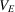and called external) generated by it over the real numbers. Its rank (n) is the minimal number of rationally independent vectors that generate the vector module. If this rank is equal to the dimension, the vector module is also a lattice. In general, a vector module of rank n and dimension m is the orthogonal projection on the m-dimensional space V of an n-dimensional lattice. We shall restrict ourselves mainly to the case m = 3 and n = 4, but the following definitions are valid for modulated phases of arbitrary dimension and rank. The dimension of the modulation (d) is nm. The modulation phases span a d-dimensional space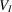(called internal or additional).

• [ii] Superspace.is an n-dimensional Euclidean space that is the direct sum of an m-dimensional external space V (of the crystal) and a d-dimensional internal space(for the additional degrees of freedom). V is sometimes denoted by.

• [iii] Split basis. For the space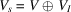, this is a basis with m basis vectors in V and d = nm basis vectors in.

• [iv] Standard basis. For the (m + d)-dimensional space, a standard basis in direct space is one having the last d basis vectors lying in(d = dimension of= dimension of the modulation). A standard basis in reciprocal space (V* identified with V) is one with the first m basis vectors lying in V (m = dimension of V).

• [v] Conventional basis. For a lattice Λ in three dimensions, it is a basis such that (i) the lattice generated by it is contained in Λ as a sublattice and (ii) there is the standard relationship between the basis vectors (e.g. for a cubic lattice a conventional basis consists of three mutually perpendicular vectors of equal length).

The lattice Λ is obtained from the lattice spanned by the conventional basis by adding (a small number of) centring vectors. [For example, the b.c.c. lattice is obtained from the conventional cubic lattice by centring the unit cell with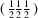.] The reciprocal basis for the conventional basis is a conventional basis for the reciprocal lattice Λ*.

In the (m + d)-dimensional superspace, a conventional basis for the latticesatisfies the same conditions (i) and (ii) as formulated above for the three-dimensional case. In addition, however, one requires that the basis is standard and such that the non-vanishing external components satisfy the relations of an (m = 3) conventional basis and that the corresponding internal components only involve the irrational components of the modulation vector(s) (for d = 1 the basis is such that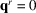, thus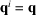). Again a conventional basis for* is dual to the same for.

• [vi] Holohedry. The holohedry of a vector module is the group of orthogonal transformations of the same dimension that leaves the vector module invariant. The holohedry of an (m + d)-dimensional lattice is the subgroup of O(m) × O(d) that leaves the lattice invariant.

• [vii] Point group. An (m + d)-dimensional crystallographic point group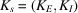is a subgroup of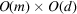. With respect to a standard lattice basis its elements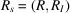are of the form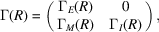where all the entries are integers and R is an element of an m-dimensional point group K, which is actually the same as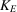. For an incommensurate modulated crystal,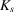and K are isomorphic groups. If d = 1,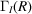== ±1.

• [viii] Geometric crystal class. Two point groupsand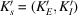of pairs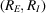of orthogonal transformations [belongs to O(m) andto O(d)] are geometrically equivalent if and only if there are orthogonal transformations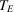and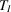of O(m) and O(d), respectively, such that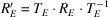and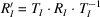for some group isomorphism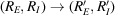. For d = 1, that condition takes a simpler form because== ±1.

• [ix] Arithmetic crystal class. A group of integral matrices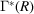[for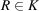of O(m)] is determined on a basis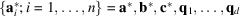of a vector module in reciprocal space by an m-dimensional point group K (here m = 3). For modulated crystals, the transformations in direct space are given by matrices Γ(R) = transpose of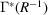which are of the form (9.8.4.17). Two groups Γ′(K′) and Γ(K) are arithmetically equivalent if and only if there is an (m + d)-dimensional matrix S of the form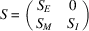with integral entries and determinant ±1 such that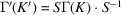. Here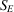is m × m and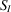is d × d dimensional. An alternative formulation is: the matrix groups Γ(K) and Γ′(K′) determined as in equation (9.8.1.16)or in equation (9.8.1.21)are arithmetically equivalent if

 (a) the groups K and K′ are geometrically equivalent m-dimensional point groups [the corresponding (m + d)-dimensional point groupsand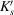are then also geometrically equivalent]; (b) there are vector module bases a*,, qd and a*′,,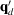such that K on the first basis gives the same group of matrices as K′ on the second basis.

• [x] Bravais class. Two vector modules are in the same Bravais class if the groups of matrices determined by their holohedries are arithmetically equivalent. Two (m + d)-dimensional lattices are in the same Bravais class if their holohedries are arithmetically equivalent. In both cases, one can find bases for the two structures such that the holohedries take the same matrix form. In the (m + d)-dimensional case, the lattice bases both have to be standard.

• [xi] Superspace group. An (m + d)-dimensional superspace group is an n-dimensional space group (n = m + d) such that it has a d-dimensional lattice of internal translations. (This latter property reflects the periodicity of the modulation.) It is determined on a standard lattice basis by the matrices Γ(R) of the point-group transformations and by the components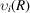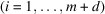of the translation parts of its elements. The matrices Γ(R) represent at the same time the elements R of the m-dimensional point group K and the corresponding elementsof the (n + d)-dimensional point groups. Two (m + d)-dimensional superspace groups are equivalent if there is an origin and a standard lattice basis for each group such that the collection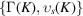is the same for both groups. [In previous formulae,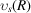is often simply indicated as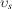.]